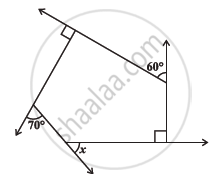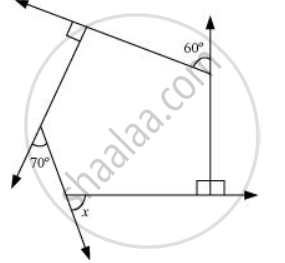# Find X In the Following Figures. 1 - Mathematics

Find x in the following figures.#### Solution60° + 90° + 70° + x + 90° = 360°

310° + x = 360°

x = 50°

Concept: Angle Sum Property of a Quadrilateral
Is there an error in this question or solution?

#### APPEARS IN

NCERT Class 8 Maths